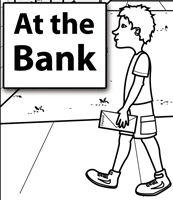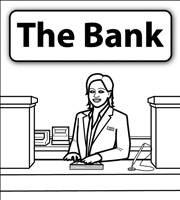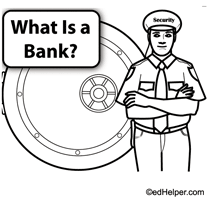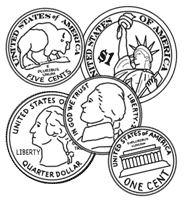edHelper.com Life Skills Writing Checks Math Worksheets Money Worksheets, Printables, Activities, and Lesson Plans 1st Grade MoneyWorksheets, Lessons, and Printables

Counting Coins: All Coins Used
US Dollar:  penny, nickel, dime, quarter, half-dollar
Canadian Dollar:  penny, nickel, dime, quarter, 50-cent piece, loonie, toonie
Euro:  one-cent, two-cent, five-cent, ten-cent, twenty-cent, fifty-cent, one-euro, two-euro

Counting (each problem only uses one type of coin)
Counting pennies
Counting nickels
Counting dimes
Mixed counting
Mixed counting multiple choice
Total value of coins

Nickels and Pennies
Counting
Multiple choice
Write total value (3-9 coins)
Write total value (7-12 coins)
Circle the coins that match the price
Draw a certain number of cents using a given number of coins
Fill in the missing numbers

Dimes and Pennies
Counting
Multiple choice
Write total value (3-9 coins)
Write total value (7-12 coins)
Circle the coins that match the price
Draw a certain number of cents using a given number of coins
Fill in the missing numbers

Pennies, Nickels, and Dimes
Counting
Multiple choice
Write total value (3-9 coins)
Write total value (7-12 coins)
Circle the coins that match the price
Draw a certain number of cents using a given number of coins
Fill in the missing numbers

Review
Money Review: Pennies, Nickels, and Dimes

Pennies, Nickels, and Quarters
Counting
Multiple choice
Write total value (3-9 coins)
Write total value (7-12 coins)
Circle the coins that match the price
Draw a certain number of cents using a given number of coins
Fill in the missing numbers

Pennies, Nickels, Dimes, and Quarters
Counting
Multiple choice
Write total value (3-9 coins)
Write total value (7-12 coins)
Circle the coins that match the price
Draw a certain number of cents using a given number of coins
Fill in the missing numbers

Review
Money Review: Pennies, Nickels, Dimes, and Quarters

Book Units
100 School Days (Grades K-2)

Money
Leveled BooksAt the BankThe BankWhat Is a Bank?American Coins

Money

Have a suggestion or would like to leave feedback?